# Pólya theorem

(diff) ← Older revision | Latest revision (diff) | Newer revision → (diff)
Jump to: navigation, search

Letbe the set of mappings from a finite set,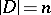, into a set, and letbe the permutation group for. This group generates a decomposition ofinto equivalence classes in which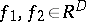belong to the same class if and only if one can find asuch that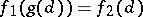for all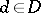. Assign to each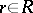a weightthat is an element of a commutative ring. The weight ofis taken to be equal to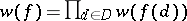and the weight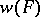of a classis defined as the weight of any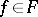. One then has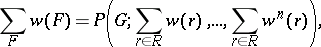where the sum on the left is taken over all the equivalence classes andis the cycle index of, where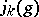is the number of cycles of lengthof the permutationin its decomposition as a product of independent cycles.

The theorem was published in 1937 by G. Pólya .

If for the weights of the elements ofone takes powers of an independent variable(or the product of powers of several variables), then for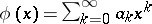(the so-called "series that enumerates figures" , where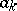is the number of elements inof weight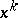) and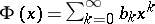(the so-called "series that enumerates configurations" , where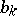is the number of classes in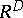of weight), the following applies, according to Pólya's theorem:### Examples.

1) If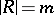,, thenand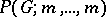is the number of equivalence classes.

2) If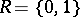,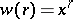, then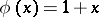, and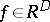withcan be interpreted as a subset ofof cardinality. The groupdefines orbits of subsets of, and the coefficient ofin the polynomial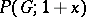is the number of orbits consisting of subsets of cardinality.

3) Letand letbe all-subsetsof a set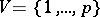; thenis a labelled graph with vertices fromin which the two verticesandare adjacent if. Let; then if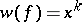,is the number of edges in the graph corresponding to the mapping. If the symmetric groupacts on, then on defining for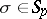the substitution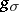inby the relation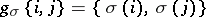one obtains a binary group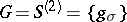acting on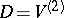.

For the sequence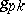(the numbers of graphs withvertices andedges),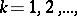Pólya's theorem gives the generating functionFor the identity permutation group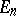, the symmetric permutation group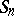and the binary permutation group, the cycle index has the form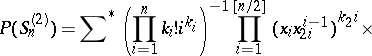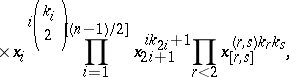respectively, whereis the greatest common divisor andis the least common multiple ofand, and the summation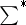extends oversubject to the condition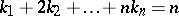. Cycle indices are known for alternating, cyclic and dihedral groups, as well as formulas for deriving the cycle indices for the product, the Cartesian product and the wreath product of groups .

There are extensions of Pólya's theorem to cases of a different definition of the weight function and equivalence classes .